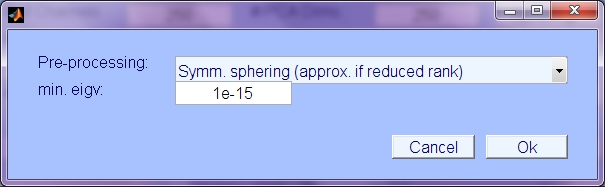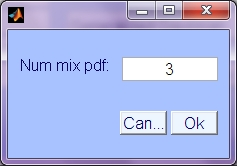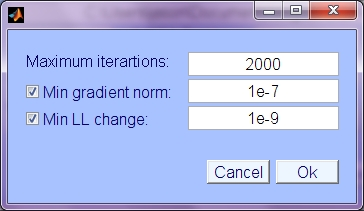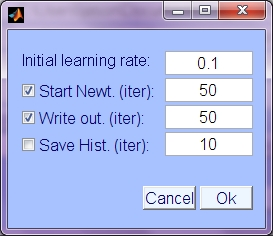# Amica 1.5 EEGLAB GUI Interface Help

This guide will describe the GUI interface for the Amica 1.5 plugin for EEGLAB, and describe the input arguments / settable keyword input:This is the main that Amica GUI window that appears when you choose Tools->Run Amica, or >> pop_runamica15(EEG). For standard purposes, none of the default arguments should need to be changed.

### Main arguments:

• In File: Name of the floating point data file (default is EEG.datfile). Keyword 'infile'.
• Out Directory: Name of the directory to write Amica output (may or may not exist). Keyword 'outdir'.
• # of Channels: Number of channels in the data (EEG.nbchan). Keyword 'num_chans'.
• # of PCA Dims: Reduce dimensionality of data to preset dimension (default EEG.nbchan). Dimension is also controlled by the mineig parameter (see Sphering description below.) Keyword 'pcakeep'.
• # of Models: Number of ICA models to estimate. See output description below. Keyword 'num_models'.
• # of Threads: For use on multi-core SMP processors. Number of threads (per nodei if using mpi). Keyword 'max_threads'.

### Sphering:• Pre-processing: Type of pre-processing (i.e. decorrelation or whitening); choices are:
• Symmetric sphering: The data is pre-whitened with the inverse of the symmetric square root of the covariance matrix. If PCA dim. is less than # chans, then pre-whitening is multiplied by the eigenvectors of a reduced dimensional subset of the principle component (eigenvector) subspace, resulting in a whitening/decorrelating, dimensional reduction that is closer to the original data space, presumed to have more intrinsic independence than the eigenvector projection (principle component activations).
• Principle Components (Eigenvectors): Use only eigenvector projection and scaling to do sphering (not symmetric or approximately symmetric)
• No sphering transformation: Only the marginal channel variances will be normalized (and mean removed) as pre-processing.
• Keword 'do_sphere' (value 1,2, or 3).
• min. eigenvalue: Value to use in determining the intrinsic data dimension. Any principle component dimensions with variance less than mineig will be discarded.

### Models:• Num. mixture pdf: Number of base component densities to use in the mixture models approximating each source density. Default is 3. Higher gives better density fit, but is slower, and prone to over-fitting if insufficient number of samples.

### Reject:• Reject Un-likely Data Samples: Use early data sample likelihoods to exclude unlikely samples from the data used for ICA optimization.
• Num. Reject: Number of times to perform rejection of unlikely data
• Num LL Std. Dev.: The number of standard deviations below the average log likelihood, below which to consider data unlikely and exclude.
• Start iter: Iteration to do first rejection of unlikely data
• Iterations between rejections: Interval in iterations between rejections, during which likelihood estimates continue adapting.

### Stopping:• Maximum iterations: maximum number of iterations to perform, regardless of other stopping conditions.
• Min gradient norm: Use norm of weight update (weight change, independent of lrate/step size) to determine stopping condition. Stop when nd (norm of delta) is less than this value. Keywords 'use_grad_norm', 'min_grad_norm';
• Min LL Change: Minimum change in Log Likelihood (LL). When LL is only increasing by this value (average over 5 iterations) then stop. Keywords 'use_min_dll', 'min_dll'.

### Lrates, etc.:• Intial learning rate: Optimizatoin begin using natural gradient update, with this initial learning rate.
• Start Newt.: Use Newton update, beginning at this iteration to ramp up to step size 1, Newton update. Since Newton update relies on estimates of (also adapting) source density parameters, the Newton update is not used until source density is accurate and Hessian is positive definite. Currently this is set by default to 50, which has been found to work well generally. Keywords 'do_newton', 'newt_start'
• Write output: Iteration interval at which to write the output to the output directory (so that it can be monitored at snapshots during the optimization process). Keyword 'writestep' (set to 0 to disable preliminary writing).
• Save History: Save history of updates, writing snapshots to outdir/history/ folder at intervals of this number of iterations. Keywords, 'do_history', 'histstep'.

### Block sizes:• Matrix Block Size: Data is processed in chunks (blocks) for efficiency and buffer size limitation reasons. Use this block size if not optimizing. Keyword 'blocksize'
• Optimize Block Size: Try several block sizes on standard data computation and choose the one with fastest iteration time. Keyword 'do_opt_block'.
• Min block size: In block size optimization, start testing at this block size.
• Block test step: Increase from minimum block size in this size increments.
• Max. block size: Do not test block sizes greater than this.

### MPI: